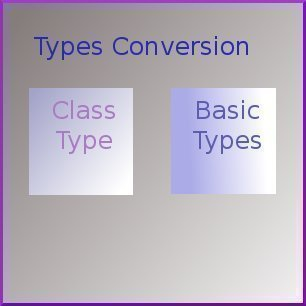•• # Type Conversion – Class to Basic TypeThe constructor handles the task of converting basic types to class types very well. But you cannot use constructors for converting class types to basic data types. Instead, you can define an overloaded casting operator that can be used to convert a class data type into a basic data type. The general form of an overloaded casting operator function is shown below. This function is also known as a conversion function.

Syntax:

.cf { font-family: Lucida Console; font-size: 9pt; color: black; background: white; }
.cl { margin: 0px; }
.cb1 { color: green; }
.cb2 { color: blue; }
.cb3 { color: maroon; }

`operator typename()`
`{`
` -----------`
` function body`
` -----------`
`}`

The above function converts the class type into the typename mentioned in the function. For example, operator float( ) converts a class type to float, operator int() converts a class type to int, and so on.

Consider the following conversion function:

`Vector :: operator double()`
`{`
` double sum = 0;`
` for(int i=0; i<size; i++)`
` sum += v[i] * v[i];`
` return sqrt(sum);`
`}`

The above function converts a Vector object into its corresponding scalar magnitude, in other words, it converts a Vector type into a double. [The magnitude of a vector is the square root of the sum of the squares of its components.]

The above conversion function can be accessed in either of two ways:

1. double length = double(V1); OR
2. double length = V1;

In the above statements, V1 is an object of the Vector class. Statements 1 and 2 perform the same task of converting a class type object to a basic data type. Whenever the compiler encounters similar statements it automatically calls the casting operator function to convert the class type to a basic data type.

The casting operator function should satisfy the following conditions:

• It must be a class member.
• It must not specify a return type.
• It must not have any arguments. The object that invokes this function has its members directly accessible since this is a member function.

## Sample Program

`/*`
`* Program that converts between kilometers and miles.`
`* Illustrates type conversion from a class type`
`* to a built-in type.`
`* https://www.tech-faq.com`
`*/`
`#include<iostream>`
`using namespace std;`
`class DistConv`
`{`
`private:`
` int kilometers;`
` double meters;`
` nbsp; const static double kilometersPerMile;`
`public:`
` // This function converts a built-in type (i.e. miles) to the`
` // user-defined type (i.e. DistConv)`
` DistConv(double mile) // Constructor with one`
` // argument`
` {`
` double km = kilometersPerMile * mile ; // converts miles to`
` //kilometers`
` kilometers = int(km); // converts float km to`
` //int and assigns to kilometer`
` meters = (km - kilometers) * 1000 ; // converts to meters`
` }`
` DistConv(int k, float m) // constructor with two`
` // arguments`
` {`
` kilometers = k ;`
` meters = m ;`
` }`
` // ********Conversion Function************`
` operator double() // converts user-defined type i.e.`
` // DistConv to a basic-type`
` { // (double) i.e. meters`
` double K = meters/1000 ; // Converts the meters to`
` &nsp; // kilometers`
` K += double(kilometers) ; // Adds the kilometers`
` return K / kilometersPerMile ; // Converts to miles`
` }`
` void display(void)`
` {`
` cout << kilometers << " kilometers and " << meters << " meters" ;`
` }`
`}; // End of the Class Definition`
`const double DistConv::kilometersPerMile = 1.609344;`
`int main(void)`
`{`
` DistConv d1 = 5.0 ; // Uses the constructor with one argument`
` DistConv d2( 2, 25.5 ); // Uses the constructor with two arguments`
` double ml = double(d2) ; // This form uses the conversion function`
` // and converts DistConv to miles`
` cout << "2.255 kilometers = " << ml << " milesn" ;`
` ml = d1 ; // This form also uses conversion function`
` // and converts DistConv to miles`
` d1.display();`
` cout << " = " << ml << " milesn" ;`
`}`

### Output

`2.255 kilometers = 1.25859 miles`
`8 kilometers and 46.72 meters = 5 miles`

## In this Series

171 queries in 0.552 seconds.